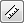# Measurement Tool

Use the measurement toolto measure distance or angle between objects in design.

Place first point anywhere in the work area. Then place the second point. Points can be selected and moved in the same way as nodes in the creation/editing mode.Panel at the right side of the screen displays measured distance and angles. (1) is distance between the points.

(2) is horizontal part of the distance (distance along the horizontal axis).

(3) is vertical part of the distance (distance along the vertical axis).

(4) is same as (1), (2) and (3), but measurements are in inches instead of centimeters.

(5) is angle between the line connecting points and horizontal axis.Measurement tool can use three points (two lines) as well. Place the 3rd point on the work area in the same way as previous points. Three points are use ful for measuring angle between objects. In such a case, (6) is this angle.

Values (1)-(5) refer to the highlighted line. (6) always refers to angle between the two lines